Formula and Execution
 Length (a) The length is the longer side of the rectangle. It is a one-dimensional quantity; so it is measured in m, cm, mm, km, ft., yds., in etc. Width (b) The width is the shorter side of the rectangle. Its dimension and units are the same as that of length. Area (A) The area is a 2-dimensional quantity that tells how much space is occupied by the rectangle. It is measured in sq. units. Perimeter (P) Perimeter is the outer boundary of a rectangle. It is the sum of all four sides of the rectangle. Perimeter being a 1-dimensional quantity, shares the same units as that of length and width. Diagonal (d) Diagonal is the line that joins the opposite corners of a rectangle together. It is also measured in 1-D units.

Area of Rectangle Calculator

Have you ever noticed your dad measuring the length and width of the living room because he has to buy a new carpet for the coming Christmas? Or have you ever noticed him measuring the wall to calculate how a big a TV screen would be most suitable? Well, these are just the very basic applications of the area of a rectangle and that too from your home. If we look around us, its applications are so vast an ocean that I can fill up pages.

Anyway, here, Calculator Beast brings for you, area of rectangle calculator to help you calculate the area of a rectangle in the flash of light.

Now whether you want to tile your floor or fence your pen for your livestock, we are just a few clicks away to help you get the most precise results for your next ventures where you need to calculate the area, perimeter, or diagonal of a rectangle shape. After all, who has time to input those measurements in complex formulas and carry out the solving process?

How to Calculate Area of Rectangle Using Calculator Beast?

With Calculator Beast’s user-friendly area of rectangle calculator, you can calculate area, perimeter, and diagonal of a rectangle by simply substituting the length and width of the rectangle in the length and width section of the calculator. And there you get precise results up to three decimal places.

An idea can be taken from the following screenshot. A rectangle of length 5 cm and width 3.5 cm is considered. On substituting these values in the calculator, we get the results for the area, perimeter, and diagonal of the rectangle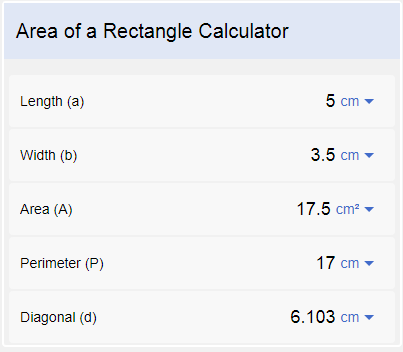Not only this, but you can also play with our area of rectangle calculator by substituting any two values to get the remaining three. Like substitute 5 cm in the length section and 17.5 cm2 in the area section and see the value of the other three occur in their respective sections.

Besides, our calculator also provides options for different units like millimeters, centimeters, kilometer, meter, inch, yard, and others. Click the drop-down arrow to see all the available units.

Rectangle Definition

Now let us learn some theory behind the rectangle, its formulas, how they work, and based on them, how our area of rectangle calculator works.

Before we move to the definition of a rectangle, let us introduce you to the term quadrilateral. A quadrilateral is a four-sided closed figure. Any shape that has four sides, four corners, and you see no open end to it, is a quadrilateral. Quadri meaning four and latus meaning sides in Latin indicate the clarity of the definition.

A quadrilateral can be of many types. One of its types is a rectangle. A rectangle is a special case of a quadrilateral that has four right angles. Its opposite sides are equal in length, and when all four sides become equal, the rectangle becomes the square. The term oblong is dedicated to a non-square rectangle, but it is occasionally used. Most of the time, the non-square rectangles are simply known as rectangles.

A rectangle is also a type of parallelogram whose angles are 900. Now, what is a parallelogram? A quadrilateral whose opposite sides are equal and parallel, but its angles between sides are not necessarily 90 degrees.

Rectangle Formulas

Rectangle formulas are those formulas that are used to measure the area of a rectangle, the perimeter of a rectangle, and the diagonal of a rectangle. Our area of rectangle calculator works on these formulas to get you your desired results.

The Area of Rectangle Formula

Consider a rectangle that has length ‘a’ units and width ‘b’ units. To find how many square units the rectangle occupies, i.e., to find the area of the rectangle, the formula used is:

A = a * b

The area is measured in square units.

The Perimeter of the Rectangle Formula

The perimeter of the rectangle is the total distance around outside the rectangle. The rectangle is 2-dimensional, but its perimeter is 1-dimensional since it is the sum of all its sides. To calculate the perimeter of a rectangle with length ‘a’ units and width ‘b’ units, the formula used is:

P = a + a + b +b

P = 2a + 2b

P = 2 (a + b)

The Perimeter is measured in 1-D units.

The Length of Diagonal of Rectangle Formula

Diagonal is a line that joins the opposite corners of the rectangles. This line divides the rectangle into two triangles, and when both the diagonals are drawn, the rectangle is divided into four triangles. To find the length of the diagonal of the rectangle with ‘a’ units length and ‘b’ units width, we use Pythagoras Theorem. The formula is:

d2 = a2 + b2

d = √( a2 + b2)

Diagonal is also a 1-dimensional quantity and it is measured in the units of length and width of the rectangle.

Examples

1. Find the area, perimeter, and length of diagonal of a rectangle whose length and width are 22 cm and 15.5 cm respectively.

Let the area of the rectangle be ‘A’ sq. units, length be ‘a’ units, and width be ‘b’ units.

A = a*b

A = 22 * 15.5

A = 341 cm2

Let the Perimeter of the given rectangle be ‘P’ units.

P = 2(a +b)

P = 2 (22 + 15.5)

P = 75 cm

Let the length of diagonal of the rectangle be ‘d’ units.

d = √(a2 + b2)

d = √(222 + 15.52)

d = 26.912 cm

The above calculation will take you quite some time. With our online area of rectangle calculator, just substitute the values of length and width in their respective sections and ta-da!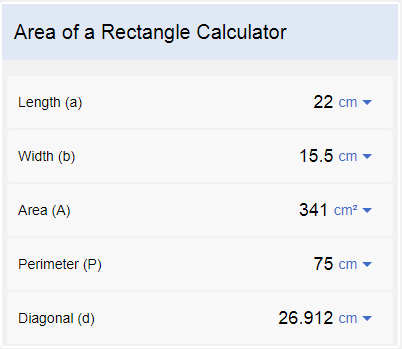2. The perimeter of a rectangle is 11cm, and its width is 4cm. Find its area.

Let the length of the rectangle be ‘a’ units, width be ‘b’ units, and perimeter be ‘P’ units.

P = 2(a +b)

11 = 2(a + 4)

2a + 8 = 11

2a = 11 – 8

a = 1.5cm

Let the area of the rectangle be ‘A’ sq. units.

A = a * b

A = 1.5 * 4

A = 6 cm2

To save yourself from this much of work, just enter the values of perimeter and width in their respective sections and get the other results magically!

Yes, our area of rectangle calculator works that way too. You do not need to have length and width every time. Any values from any of the values of area, perimeter, or diagonal length with one value of either dimension of the rectangle would yield other results. Amazing, right?

Note: Make sure to use the correct unit.

FAQs

1. What are the properties of a rectangle?

A rectangle is very interesting when it comes to knowing its properties. Rectangles have perfect reflectional symmetries, and when rotated around an axis, they exhibit rotational symmetry too. Some of the properties of a rectangle are:

• It is a quadrilateral with all interior angles equal (equiangular property).
• Since the sum of the interior angles of a polygon 360 degrees, the interior angles of a rectangle are 90 degrees (360/4).
• The opposite sides of a rectangle are equal and parallel.
• The diagonals of a rectangle bisect each other, i.e., they intersect one another exactly from the center.
• Both the diagonals are equal in length.
• The diagonals of a rectangle bisect one another at different angles. One is the obtuse angle, and the other is acute.
• If the angle of the bisection of a rectangle is 90 degrees, it is a square.
• The point of intersection of diagonals of a rectangle is the center of the circumcircle (a circle that passes through all four corners of a rectangle) whose radius is half of the length of the diagonal (cyclic property).
• When the rectangle is a square, an incircle can be drawn with the bisection point of diagonals as the center of the circle.
• Note: no incircle can be drawn in an oblong.
• When we join the midpoints of the opposite sides, four rectangles are formed within. And when we join the diagonals together, four triangles are formed within. But no squares are formed even after the fact that all four angles of the rectangle are 900.
• The triangles formed within the rectangle by the bisection of diagonals are isosceles triangles.
• Every rectangle is a parallelogram, but every parallelogram is not a rectangle.
• Every square is a rectangle, but every rectangle (oblong) is not a square.
• Every rectangle is a trapezoid, but every trapezoid is not a rectangle.
• A rhombus can be drawn within the rectangle by joining the midpoints of the sides such that they are parallel to the diagonal. The rhombus formed has half the area of the rectangle.
• When a rectangle is rotated along the longer side, a cylinder is obtained whose length is equal to the length of the rectangle, and the diameter is equal to twice the size of the width of the rectangle.
• When a rectangle is rotated along the shorter side, a cylinder is obtained whose length is equal to the width of the rectangle, and the diameter is equal to twice the size of the length of the rectangle.
• When a rectangle is rotated along the line joining the midpoints of the shorter sides, a cylinder is obtained whose length is equal to the length of the rectangle, and the diameter is equal to the width of the rectangle.

Can you think of any more properties that a rectangle exhibits?

2. What is the Golden Ratio?

A golden ratio is the ratio of sides of a special rectangle known as a golden rectangle. If you cut off a square from the rectangle, the leftover rectangle has the same proportion as the original rectangle, i.e., a+b/a = a/b = φ = 1.618

Here φ is the golden ratio. The figure illustrates the idea of what a golden rectangle looks like.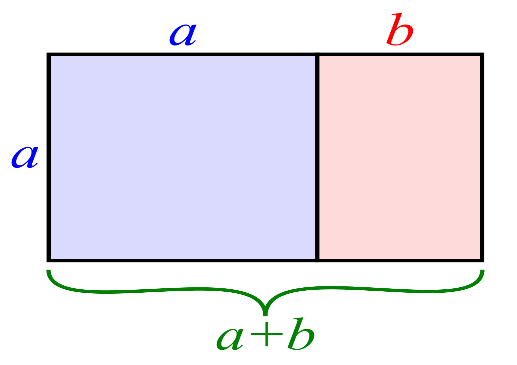It has been claimed that the golden rectangle appears in ancient Greek architecture and some famous artwork, but this is not carried out by measurements. Look at the pictures below, and see how beautifully the golden ratio is incorporated into them.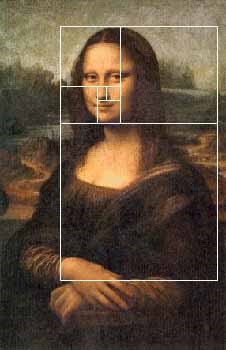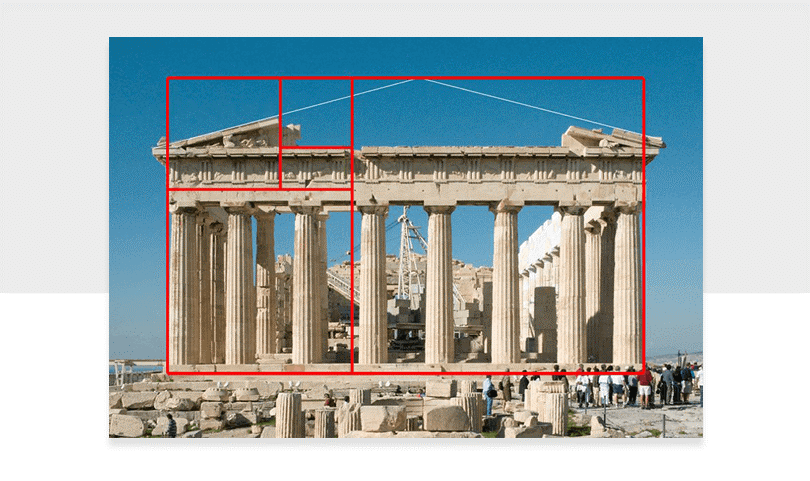You must be thinking why I said it would blow your mind. So, here is the answer to that.

The golden ratio exists everywhere in nature. Consider a golden rectangle. If we start making incremental golden rectangles within this rectangle, we see the golden spiral form.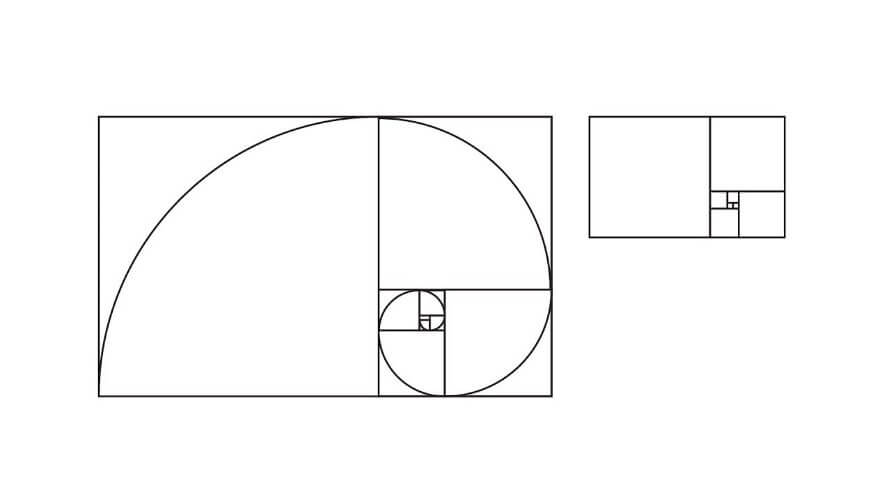Now, this has to do a lot with your daily life. If we see nature, we see many things flourish when they follow the golden ratio design. If plants are grown to the ratio of golden ratio spiral, the maximum amount of rain is directed down to the roots.

This ratio is also the mathematical sequence in the spirals of storms, tornadoes, and hurricanes.

The baby in a mother’s womb forms a golden spiral; your ears form a golden spiral too. The human body follows this golden ratio in every way. Even the rhythmic pattern of your heartbeat follows this golden ratio.

The spiral of the Milky Way galaxy, the way the celestial bodies are arranged in the universe, and many other planets follow this golden ratio.

It does not end here. The golden ratio also comes up in the ancient Biblical texts.

Painters and architects use this golden ratio to design their paintings and sculptures for perfection. Designs tend to look so much better when they follow the golden ratio.

The golden ratio is embedded into our lives so much. It affects our arts and aesthetics. It is used in the product design, in logos, and in branding all the time.

This ratio, for some reason, is pleasing to the eye.

Now go out and look around yourself and see where you can find the golden ratio. I bet you will see it everywhere.

Mind-blowing, isn’t it?

3. What are incomparable rectangles?

Rectangles are said to be incomparable rectangles when two rectangles do not fit inside one another.

4. For what condition, the area of a rectangle, is the same as its perimeter?

When the area and perimeter of the rectangle with length ‘a’ and width ‘b’ are same, we have the following condition:

ab = 2 (a + b)

ab = 2a + 2b

ab – 2a = 2b

a (b-2) = 2b

a = 2b/(b-2)

For the above relation, the area and perimeter of a rectangle are the same. The above condition works for all real numbers except b=2. At this value, the denominator becomes zero, and division by zero is undefined. Also, if 0< b<2, the value of ‘a’ becomes less than zero, and length can never be negative. So to make sure that the above relation is neither undefined nor generates any negative value for length, we must have b>2.

5. What rectangular patterns are used for tessellation?

We see rectangular tessellation everywhere around. From our floor tiles, wall tiles to sidewalks. Some of the tessellation patterns are:

·        Stacked bond

·        Running bond

·        Herringbone pattern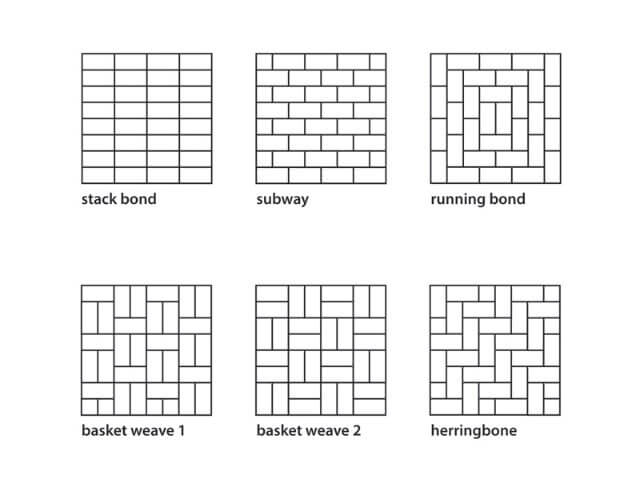6. What are some facts about the rectangle?

• We are surrounded by rectangular shape more than any other shape. Now just look around yourself and list the things that you see and name their shape. Now calculate how many objects are rectangular and compare them to shapes of the other things. Rectangle won, didn’t it?
• Take a rectangle cloth, like, say a sheet. Now fold the sheet such that the opposite sides meet together (like everyone normally does). Keep on folding the sheets in a similar fashion. You cannot fold it more than seven times in total. Try out with every rectangle cloth or paper you see, no matter how big or small it is. Tried? Mysterious, right?
• People always come up with new and creative ideas to rank things. Like the mighty earth has been divided into larger continents and then smaller countries. They have then found ways to rank them. Like China stands first when it comes to population, the US has the largest economy, Canada takes the list in education, Russia wins in area, and many such rankings exist.
• But have you ever thought about the ranking of the most rectangular country? Yes, strange, right? But the ranking exists. And the winner is Egypt. Like, not that the countries are exactly rectangular, but their extent to rectangularity has been calculated by David Barry, an Australian geo-statistician. A similar ranking exists for the roundness of the countries. And guess what? The Vatican is the 4th-roundest yet the 2nd-rectangular country. Perplexed, aren’t you? Now go and look at the map.

It is Fun to Know!

Have you ever wondered why your cellphones are rectangular in shape? Well, you are so much used to of that device, it never occurred to you that they could have been circular or square in shape too, but they aren’t. That is because a rectangle is what you want them to be.

A rectangle is the most convenient shape for a screen. You can flip your phone because of its orientation, and it will adapt either horizontal or vertical orientation as per your preference. Imagine flipping a rounded phone. Whoa, I already feel dizzy. It has no orientation, and you would be left with zero choices to rotate your phone. The same goes for the square. Like, with all sides equal, you wouldn’t have a choice here either to rotate the screen.

Well, rotating the screen isn’t just the only thing. The other reason is to carry them. It is far easier to carry a rectangular shaped phone in your pocket than a squared or rounded phone. A pocket is rectangular, and so is your phone.

It is easy to handle a rectangle shape in your palm than a round or square or any other shape. Besides, our eyes are used to having rectangular objects around us than any other shape. Books, papers, photographs, doors, windows, tabletops, cushions, pools, mirrors, tessellation patterns, and what not?

By the way, attempts to make rounded and squared phones have been made, but yes, those shapes did not get much success.

So, can you think of other things that are rectangular and why they are rectangular?

For me, the answer is quite simple. May be humans always knew the elegance and convenience of a rectangle shape!